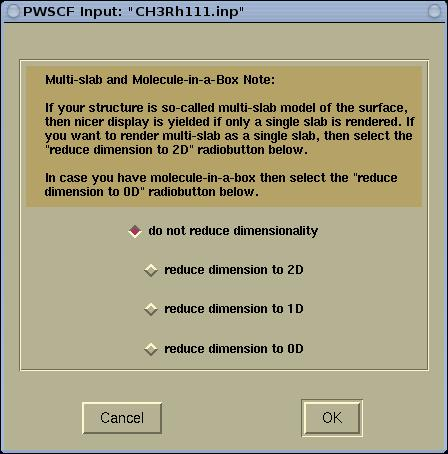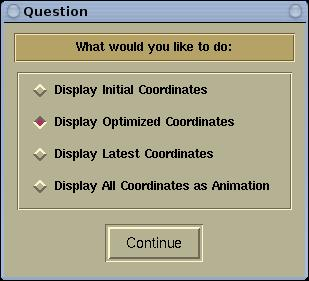XCrySDen as a graphical tool for Quantum ESPRESSO

# Displaying the crystal structure from the pw.x Input/Output files.

XCrySDen can display the crystal (or molecular) structures from the PWscf input and output files (here the pw.x code is meant).

Before visualizing the structure, the program will query for possible reduction of the structure's dimension (here periodic dimensions are meant). For example, molecule has zero periodic dimensions, while surface (slab) has two periodic dimensions, therefore it is recommended to display them with the appropriate number of periodic dimensions.

Here is the periodic query window:## The pw.x Input file

The crystal (or molecular) structure from the pw.x input file can be displayed either by the appropriate command line option or from the menu.

From command-line:

• either: xcrysden --pwi file
• or: xcrysden --pw_inp file
Frome menu: select the menu item File-->Open PWscf ...-->Open PWSCF Input File

## The pw.x output file

The crystal (or molecular) structure from the pw.x input file can be displayed either by the appropriate command line option or from the menu.

From command-line:

• either: xcrysden --pwo file
• or: xcrysden --pw_out file
Frome menu: select the menu item File-->Open PWscf ...-->Open PWSCF Output File

There are four types of coordinate extraction modes, and this will be queried with the following window:Below is the description of the four types of extraction modes.

### The initial structure

The Display Initial Coordinates mode will display the initial coordinates printed at the beginning of the pw.x output file, i.e., those printed in the following manner:
```
site n.     atom                  positions (a_0 units)
1           H   tau(  1) = (  -.1657590  -.0955104  1.2559945  )
2           H   tau(  2) = (   .1659009  -.0954643  1.2557377  )
3           H   tau(  3) = (   .0000000   .1917322  1.2548077  )
4           C   tau(  4) = (   .0000000   .0000000  1.1886906  )
5           Rh  tau(  5) = (   .0000000   .0000000   .8054534  )
6           Rh  tau(  6) = (   .7499998  -.4330126   .8054534  )
7           Rh  tau(  7) = (   .4999998   .0000000   .8054534  )
8           Rh  tau(  8) = (   .2499999  -.4330126   .8054534  )
9           Rh  tau(  9) = (  -.2499999   .1443376   .4082482  )
10           Rh  tau( 10) = (   .0000000  -.2886750   .4082482  )
11           Rh  tau( 11) = (   .4999998  -.2886750   .4082482  )
12           Rh  tau( 12) = (   .2499999   .1443376   .4082482  )
13           Rh  tau( 13) = (  -.4999998   .2886750   .0000000  )
14           Rh  tau( 14) = (  -.2499999  -.1443376   .0000000  )
15           Rh  tau( 15) = (   .2499999  -.1443376   .0000000  )
16           Rh  tau( 16) = (   .0000000   .2886750   .0000000  )
```

### The optimized structure

The Display Optimized Coordinates mode will display the optimized coordinates, therefore this mode is useful only for the output file of geometry optimizations. If the optimized coordinates are not found in the output file, the program will signal an error. The optimized coordinates are printed in the following manner in the output file:
```
End of BFGS geometry calculation

Final energy            =   -1226.4092020951 ryd

Saving the approximate inverse hessian

CELL_PARAMETERS (alat)
1.000000000   0.000000000   0.000000000
0.000000000   1.414213562   0.000000000
0.000000000   0.000000000   3.150000000

ATOMIC_POSITIONS (alat)
O       -0.000066379  -0.513244840   1.345933583
N        0.000016050  -0.730745427   1.464247866
N       -0.000018075  -0.898866014   1.326587159
Pd      -0.000083375  -0.711492446   1.013218636
Pd       0.499979720  -0.706754811   0.987470168
Pd       0.000094941   0.000039404   0.983520698
Pd       0.500073136   0.000254615   0.980957462
Pd      -0.248933604  -0.357455663   0.757427630
Pd       0.248979012  -0.357447626   0.757410448
Pd      -0.248500404   0.358259400   0.758511634
Pd       0.248539175   0.358243548   0.758490039
Pd       0.000000000  -0.707106780   0.500000000
Pd       0.500000000  -0.707106780   0.500000000
Pd       0.000000000   0.000000000   0.500000000
Pd       0.500000000   0.000000000   0.500000000
Pd      -0.250000000  -0.353553390   0.250000000
Pd       0.250000000  -0.353553390   0.250000000
Pd      -0.250000000   0.353553390   0.250000000
Pd       0.250000000   0.353553390   0.250000000
Pd       0.000000000  -0.707106780   0.000000000
Pd       0.500000000  -0.707106780   0.000000000
Pd       0.000000000   0.000000000   0.000000000
Pd       0.500000000   0.000000000   0.000000000
```

### The latest structure

The Display Latest Coordinates mode will display the latest coordinates in the output file. If the latest printed coordinates are the optimized coordinates, then these will be displayed.

### All the structures

The Display All Coordinates as Animation mode will display the all the coordinates found in the output file, including the initial coordinates. This mode is useful for geometry optimizations and molecular dynamics calculations. The user will have the possibility to animate forth and back through all the "images" of the involving structure during the calculation.

# Selecting the k-path for spaghetti band structure plots

Whenever a given crystal structure is displayed by XCrySDen, it is possible to graphically select a k-path for the band structure calculation (spaghetti plot) via the Tools-->k-path Selection menu. Read here the description of how to select graphically the k-path.

IMPORTANT: to save the selected k-path in the format compatible with the PWscf, make sure to save the file with the .pwscf extension (example: my_kpath.pwscf).

# Visualizing charge densities, STM Images, and alike

To visualize charge densities, STM images, and similar properties with XCrySDen user needs to calculate this property and store the data into the XSF format. Here is the sequence of the PWscf tasks that needs to be performed:
1. the SCF calculation: pw.x < pw_input > pw_output
2. the post-processing calculation, where a given property is selected and an XSF format specified:
pp.x < pp_input > pp_output